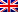# The M / M / 1 queue

Difficulty
Easy 25%

Contenus

## Queue M / M / 1

An M / M / 1 queue follows an exponential law for the arrival and service of customers. An M / M / 1 queue is represented as follows:

In the majority of cases, the customer in a service is included in the number of customers in the waiting line.

The number of customers in the queue is modeled by the markov chain following continuous time:

Stationary probabilities exist because the chain is irreducible. Denote by p (n) the probability that the number of clients in the queue N (t) = n as t tends to infinity. The equilibrium equations give the following system:

If we set ρ = λ / μ then we find p (n) = ρnotp (0), which implies:

We deduce that the queue is stable if ρ <1. That is to say that the average customer processing time is strictly less than the average arrival time of a customer (i.e. the average time between 2 customer arrivals). The queue is unstable if ρ≥1, in this case the clients accumulate ad infinitum in the queue.

All the performance parameters are calculated in steady state if the queue is stable. If we apply Little's law and the performance measures to M / M / 1 queues (and more generally to M / M / S queues), with ρ = A:FRFRENES